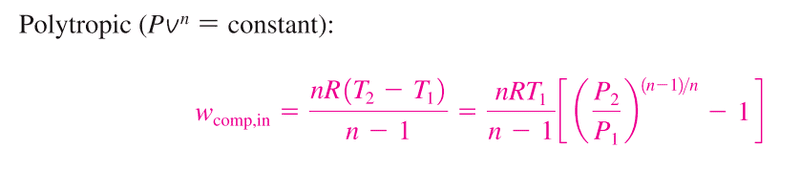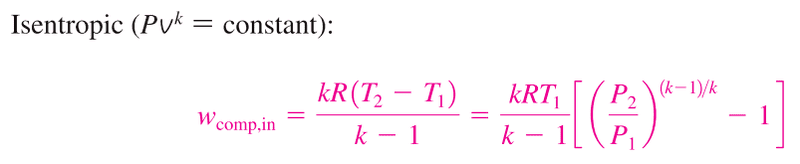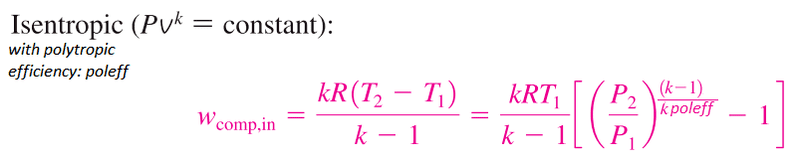# Help to understand polytropic exponent and efficiency

Hello all,

I am new to thermodynamics applied to turbines and compressors and I am trying to get my head around what is represented when calculating the work of a compression/expansion process using the polytropic exponent as oppose to the specific heat ratio of 1.4 (I'm working with air).

The attached image ( from Çengel, Y. a. (2004). Thermodynamics: An Engineering Approach. McGraw-Hill.)

Shows the equations for polytropic compression.So I have two questions:
1) is the polytropic equation taking into account any heat losses compared to the following isentropic equation:At the moment I am using the isentropic formulae and dividing it by the isentropic effciency which gives me a work value slightly higher accounting for irreversibilities.

2) What is the difference between using the polytropic exponent, n. Like in the first equation. And using the polytropic efficiency as below:The reason I am asking is because using the polytropic exponent, n gives me greater efficiencies for my compression and expansion system than the ratio of specific heats, k. i.e lower compressor work and greater turbine work. Which I wouldn't expect if the polytopic exponent accounted for losses.

Thank you for your help.

## Answers and Replies

Gold Member
... I have two questions:
1) is the polytropic equation taking into account any heat losses compared to the following isentropic equation:View attachment 99520
At the moment I am using the isentropic formulae and dividing it by the isentropic effciency which gives me a work value slightly higher accounting for irreversibilities.

2) What is the difference between using the polytropic exponent, n. Like in the first equation. And using the polytropic efficiency as below:
View attachment 99521
The reason I am asking is because using the polytropic exponent, n gives me greater efficiencies for my compression and expansion system than the ratio of specific heats, k. i.e lower compressor work and greater turbine work. Which I wouldn't expect if the polytopic exponent accounted for losses.

Thank you for your help.

To part 1, a polytropic process assumes that during compression/expansion, the ratio of work being done to heat being transferred is a constant. One example is when air is compressed in an engine's cylinder. There is work being done on the gas, and as it heats up, it transfers heat out of the cylinder. The P-V diagram typically suggests a polytropic expansion coefficient of 1.3. The catch (in my mind) is that it's doubtful that the ratio of work to heat is constant - the cylinder walls here could be hotter than the air at one point in time, and cooler at another. I haven't given much thought about what conditions would actually cause a truly constant value of n, but I suspect they are rare.

I can't say much about part 2, as I haven't thought through a comparison of the two methods you described.

Mentor
In a polytropic process, heat is being exchanged with the surroundings in such a manner that the exponent n remains constant. In an adiabatic reversible process, no heat is exchanged with the surroundings. So, in a polytropic process, you need to determine Q, the heat transferred to the system, and take that into account in your efficiency analysis.

•